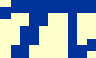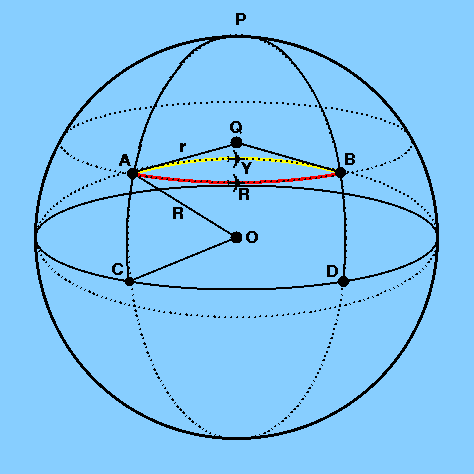1 The latitude and longitude of Sheffield are 53°23´12´´ N and 1°28´07´´ W, respectively. The latitude and longitude of Sydney are 33°55´24´´ S and 151°17´03´´ E, respectively. What is the difference in the latitude of the two cities in decimal degrees? Difference in latitude = 53°23´12´´ - ( - 33°55´24´´) = 87°18´36´´ Difference in latitude = 87° + (18/60)° + (36/3600)° = 87.3100° What is the difference in the longitude of the two cities in decimal degrees? Difference in longitude = 1°28´07´´ - ( - 151°17´03´´) = 152°45´10´´ Difference in longitude = 152° + (45/60)° + (10/3600)° = 152.7528° What is the difference in longitude in hours, minutes and seconds of time? Difference in longitude = 24 x 152.7528 / 360 = 10.18352h Difference in longitude = 10h (0.18352 x 60)m = 10h11.0112m Difference in longitude = 10h11m (0.0112 x 60)s = 10h11m00.67s What are the co-latitudes of Sheffield and Sydney? Latitude of north pole = 90° N co-latitude of Sheffield = 90° - 53°23´12´´ = 36°36´48´´ Latitude of south pole = 90° S co-latitude of Sydney = 90° - 33°55´24´´ = 56°4´36´´

 2 Given that the mean radius of the Earth is 6370 km, convert the nautical mile and the knot into miles and mph. Circumference of the Earth = 2x 6370 km Number of arcminutes in 360° = 360 x 60 = 21600´ Length of arc subtended by 1´ = 2x 6370 / 21600 = 1.85 km Therefore, 1 nautical mile = 1.85 km = 1.85/1.61 miles = 1.15 miles and, 1 knot = 1.85 km/h = 1.15 mph.

 3 How much longer will it take to fly from Sheffield to Petropavlovsk in Russia along the parallel compared to the great circle route? Assume that Sheffield and Petropavlovsk are at the same latitude (53°23´ N), the longitude of Sheffield and Petropavlovsk are 1°28´ W and 158°42´ E, respectively, and the plane is flying at 500 knots.

 figure 8: a flight from Sheffield to Petropavlovsk in RussiaLet A and B in Figure 8 represent Sheffield and Petropavlovsk, so that the parallel route is denoted by the red arc ARB and the great circle route is denoted by the yellow arc AYB. If the meridians PAC and PBD are drawn from the north pole P through A and B to the equator CD, triangle PAYB is a spherical triangle. Applying the cosine formula, we may then write

cos AYB = cos AP cos BP + sin AP sin BP cos APB
AP = BP = 90° - 53°23´ = 36°37´ = 36.6167°
APB = 1°28´ - (- 158°42´) = 160°10´ = 160.1667°.

Substituting these numbers into the cosine formula gives

cos AYB = (cos 36°.6167)2 + (sin 36°.6167)2 cos 160.1667°
AYB = 71°.9663 = 71°58´ = 4318´.

The great circle distance between Sheffield and Petropavlovsk is therefore 4318 nautical miles and hence it will take 4318/500 = 8.636 h = 8h38m to complete the journey via the yellow arc in Figure 8.

The distance between Sheffield and Petropavlovsk along the parallel of latitude 53° 23´ N (a measurement often referred to as the departure) can be calculated as follows:

The circumference of the parallel at latitude 53°23´ N = 2r,
where r = R cos AOC, AOC = 53°23´ = 53.3833° and R = radius of the Earth = 3443 nautical miles.

The red arc ARB in Figure 8 covers only a fraction of this circumference, where the fraction is given by AQB/360° and AQB is given by the difference in longitude of A and B. So,

ARB = (160°.1667/360°) x 2x 3443 x cos 53°.3833 = 5741 nautical miles.

Hence it will take 5741/500 = 11.482 h = 11h29m to complete the journey via the red arc in Figure 8 and so the journey between Sheffield and Petropavlovsk is 2h51m quicker along the great circle route than along the parallel.